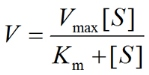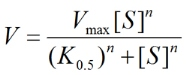PhysiologyWeb  Home  |  FAQ  |  About  |  Contact  |   - Sign InTweetPhysiology Quiz -
Michaelis-Menten Kinetics - Part 1
Michaelis-Menten kinetics is used to describe the rate of a reaction catalyzed by an enzyme as a function of the substrate concentration. This type of analysis is also commonly used to describe the rate of transporter-mediated translocation of ions and molecules across biological membranes. Test your basic knowledge of Michaelis-Menten kinetics by taking this simple quiz.
(1)   The Km (Michaelis constant) of an enzyme-catalyzed rection:
 (A)   is a measure of the turnover rate of the enzyme. (B)   is a measure of the affinity of the enzyme for its substrate. (C)   has a higher numerical value when the enzyme has high affinity for its substrate. (D)   has a lower numerical value when the enzyme has high affinity for its substrate. (E)   is the substrate concentration when the reaction rate is half of the maximum rate.
(2)   Which of the following equations is the Michaelis-Menten equation?
 (A)(B)(3)   Which of the following equations is the Hill equation?
 (A)(B)(4)   A higher numerical value of the Michaelis constant (Km) indicates a higher enzyme/transporter affinity for its substrate.
 True False
(5)   Assume that the reaction catalyzed by an enzyme follows Michaelis-Menten kinetics. The substrate concentration (Km, Michaelis constant) needed to reach 50% of the maximum reaction velocity (Vmax) is 25 μM. What substrate concentration is required to obtain at least 95% of the maximum reaction velocity?
 (A)   25 μM (B)   50 μM (C)   100 μM (D)   250 μM (E)   475 μM
(6)   Assume that the reaction catalyzed by an enzyme follows Michaelis-Menten kinetics. If at a substrate concentration of 100 nM, the reaction proceeds at 98% of the maximum reaction velocity (Vmax), what is the Michaelis constant (Km) for this substrate. Km is the substrate concentration needed to reach 50% of Vmax.
 (A)   2 nM (B)   5 nM (C)   10 nM (D)   50 nM (E)   100 nM